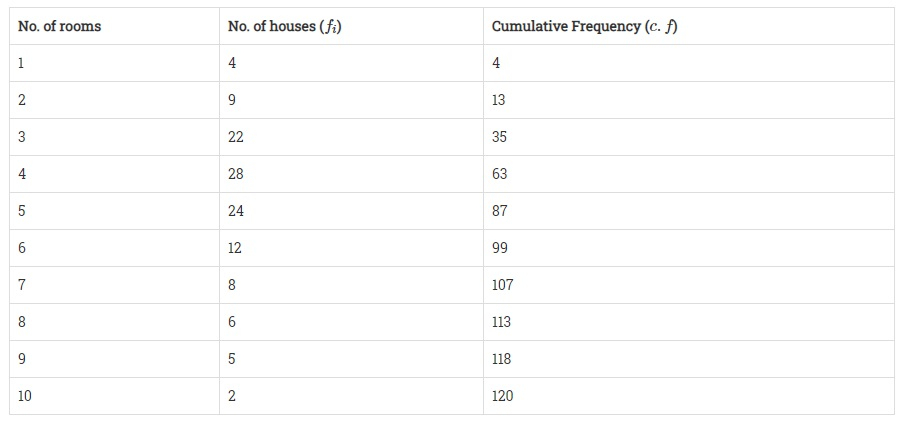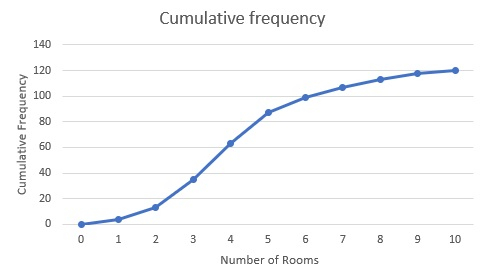# Draw an ogive by less than method for the following data:No. of rooms:12345678910No. of houses:49222824128652

To do:

We have to draw ogive by less than method for the given data.

Solution:

We first prepare the cumulative  frequency distribution table by less than method as given below:Take the number of rooms along the X-axis and cumulative frequency along the Y-axis.

Plot the points (1, 4), (2, 13), (3, 35), (4, 63), (5, 87), (6, 99), (7, 107), (8, 113), (9, 118) and (10, 120) on the graph and join them and with a free hand to get an ogive as shown. This is the required less than ogive.Updated on: 10-Oct-2022

27 Views